SSS Congruency Criteria

Congruence of Triangles
Concept wise

To prove two triangles congruent,

We use SSS Criteria – Side Side Side Criteria

In SSS Congruency Criteria,

• All 3 sides of both triangles are equal

For example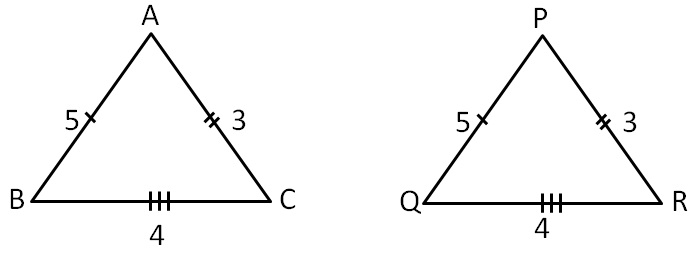Here,

All 3 corresponding sides are equal.

So, the triangles must be congruent

Let’s take some examples

### Are these triangles congruent?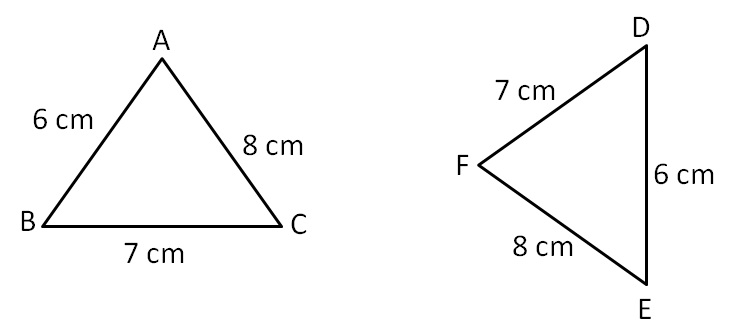In ∆ABC and ∆EDF,

AB = ED     ( Both 6 cm )

BC = DF     ( Both 7 cm )

AC = EF     (Both 8 cm )

∴ ∆ABC ≅ ∆EDF    ( SSS Congruence Rule)

Here,

A E

B D

C F

### Are these triangles congruent?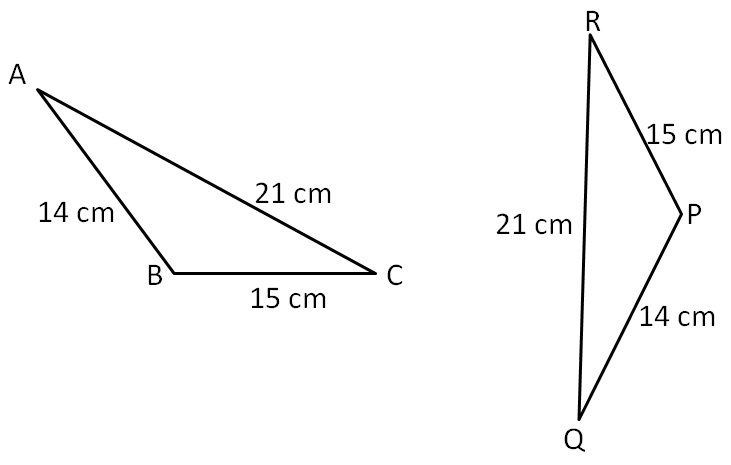In ∆ABC and ∆QPR,

AB = QP   ( Both 14 cm)

BC = PR  ( Both 15 cm )

AC = QR   ( Both 21 cm )

∴ ∆ABC ≅ ∆QPR  (SSS Congruence Rule)

Here,

A Q

B P

C R

### Are these triangles congruent?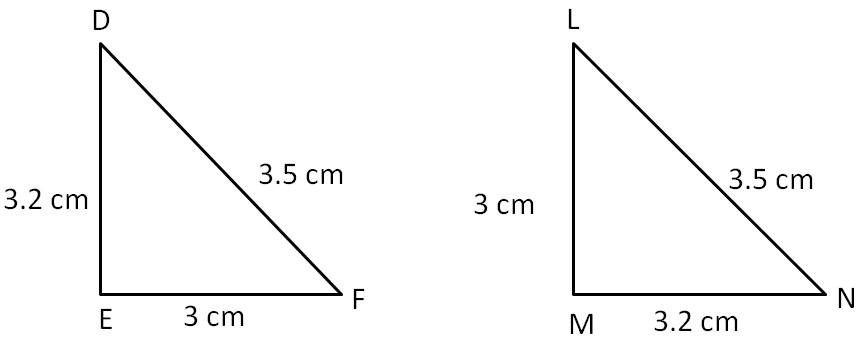In ∆DEF and ∆NML,

DE = NM  ( Both 3.2 cm )

EF = ML  (Both 3 cm )

DF = NL  ( Both 3.5 cm )

∴ ∆DEF ≅ ∆NML   ( SSS Congruence Rule)

Here,

D N

F L

E M

### Are these triangles congruent?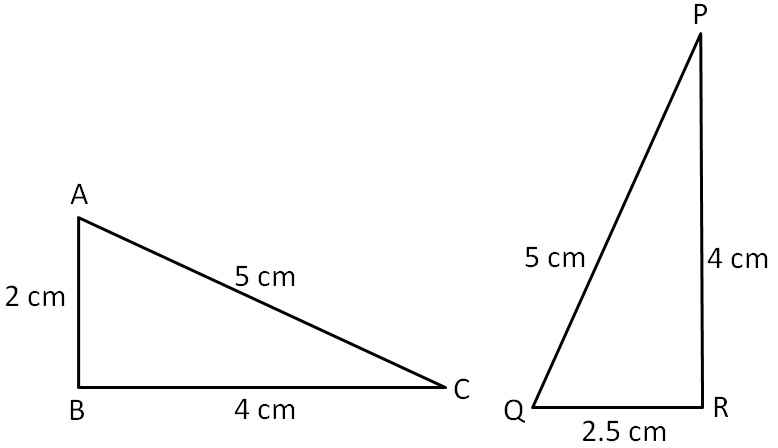In ∆ABC and ∆QRP,

AC = QP       ( Both 5 cm )

BC = RP       ( Both 4cm )

AB ≠ QR

Since all sides are not equal,

∴ ∆ABC ≇  ∆QRP

### Are these triangles congruent?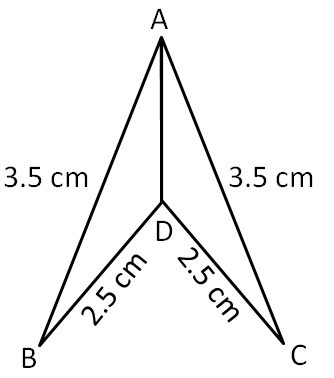AB = AC      (Both 3.5 cm)

DB = DC     (Both 2.5 cm)

Here,

A A

B C

D D

In Fig, AB =AC and D is the mid-point of (BC) ̅.

(iii)Is ∠B = ∠C? Why?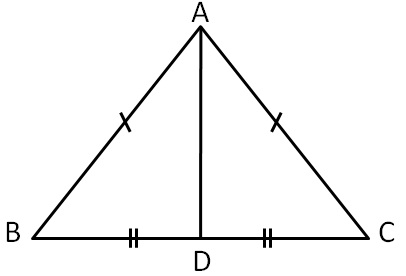AB = AC     ( Given )

DB = DC    ( D is the mid – point of BC )

Also,

∠B = ∠C      (By CPCT)

Here,

A A

B C

D D

In Fig, AC = BD and AD = BC. Which of the following statements is meaningfully written?

(i)∆ABC ≅ ∆ABD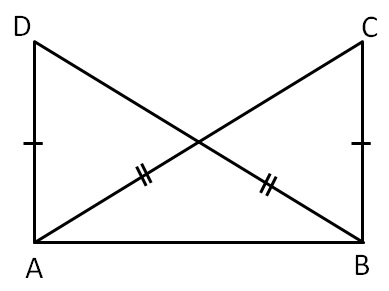AB = AB     (Common )

AC = BD     (Given )

∴ ∆ABC ≅ ∆BAD     ( SSS Congruence Rule)

Here,

A B

C D

B A

Hence (ii) is correct

Learn in your speed, with individual attention - Teachoo Maths 1-on-1 Class